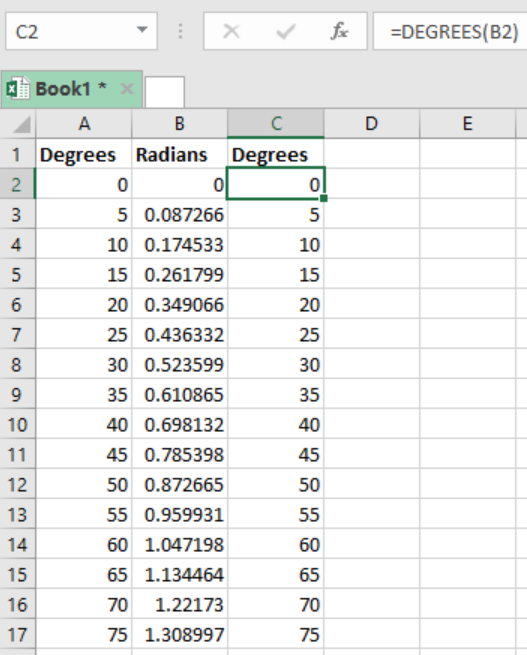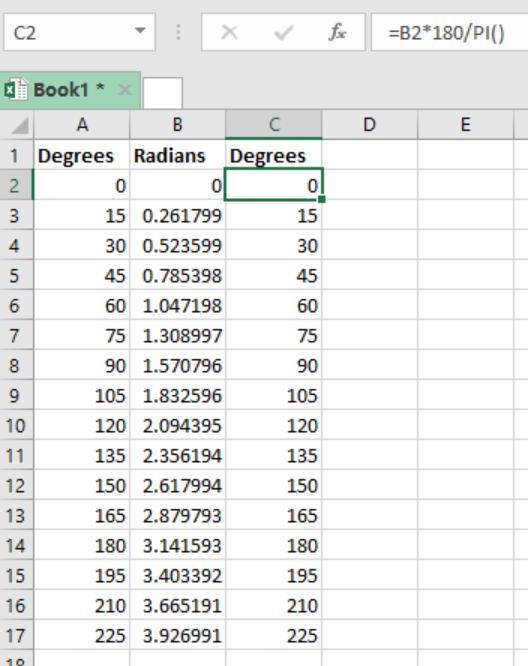Get instant live expert help with Excel or Google Sheets“My Excelchat expert helped me in less than 20 minutes, saving me what would have been 5 hours of work!”

#### Post your problem and you’ll get Expert help in seconds.

Your message must be at least 40 characters
Our professional Expert are available now. Your privacy is guaranteed.

# How to Put Excel in Degrees Mode

When working with excel spreadsheets, you might want to get the answer in degrees but the Excel is returning it in radians. How can you set the Excel to return the answer in degrees instead of radians? This post provides ways on how to put excel in degrees mode.

There are basically two ways to change radians into degrees, so as to have your answer in degrees form. Let us look at both methods

## Method 1: Use of the DEGREES function

The first methods to set excel into degrees mode is by the use of the FUNCTION. This is as illustrated in the figure below;Figure 1: How to put Excel to Degrees mode using DEGREES function

In this example, we have converted the radians in column B to degrees in column C with the help of the excel DEGREES function.

## Method 2: By help of formula

We can also change excel from radians mode to degrees mode by the use of a formula. The formula is as shown below;

`=A1*180/PI()`

Where;

• A1- refers to the cell reference with values in radians.

## ExampleFigure 2: How to put Excel into degrees mode using formula

In this example, we have used a formula to put the Excel into degrees mode. In cell C2, we have the following formula;

`=C2*180/PI()`

This will change the radians into degrees mode in excel.

## Instant Connection to an Expert through our Excelchat Service

Most of the time, the problem you will need to solve will be more complex than a simple application of a formula or function. If you want to save hours of research and frustration, try our live Excelchat service! Our Excel Experts are available 24/7 to answer any Excel question you may have. We guarantee a connection within 30 seconds and a customized solution within 20 minutes.

Solution examplesIf I merged a multi-row, multi-column selection. Iand types some text into the merged cell and then unmerges it, what will happen to the text?
Solved by S. Y. in 60 mins